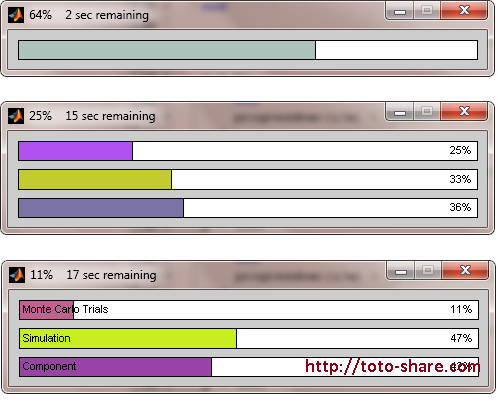# New Matlab Progressbar

From my last post, I am write about how to create Matlab Progressbar using Matlab waitbar function. In this post, I want to write how to create New Matlab Progressbar with more complete feature (colour, time to finishing computation, support multi progressbar, etc). I dont create this script, but I get this script when searhing at www.mathworks.com. This is some of figure from this New Matlab Progressbar script :We can change width and height from this New Matlab Progressbar by editing file progessbar.m at line 180 to 183. Change this line and check how your New Matlab Progressbar changed.

This is a method how to implementated this New Matlab Progressbar in our code, copy this code and try to running this code from your Matlab program :

```% Single bar
m = 500;
progressbar % Init single bar
for i = 1:m
pause(0.01) % Do something important
progressbar(i/m) % Update progress bar
end

% Simple multi bar (update one bar at a time)
m = 4;
n = 3;
p = 100;
progressbar(0,0,0) % Init 3 bars
for i = 1:m
progressbar([],0) % Reset 2nd bar
for j = 1:n
progressbar([],[],0) % Reset 3rd bar
for k = 1:p
pause(0.01) % Do something important
progressbar([],[],k/p) % Update 3rd bar
end
progressbar([],j/n) % Update 2nd bar
end
progressbar(i/m) % Update 1st bar
end

% Fancy multi bar (use labels and update all bars at once)
m = 4;
n = 3;
p = 100;
progressbar('Monte Carlo Trials','Simulation','Component') % Init 3 bars
for i = 1:m
for j = 1:n
for k = 1:p
pause(0.01) % Do something important
% Update all bars
frac3 = k/p;
frac2 = ((j-1) + frac3) / n;
frac1 = ((i-1) + frac2) / m;
progressbar(frac1, frac2, frac3)
end
end
end```